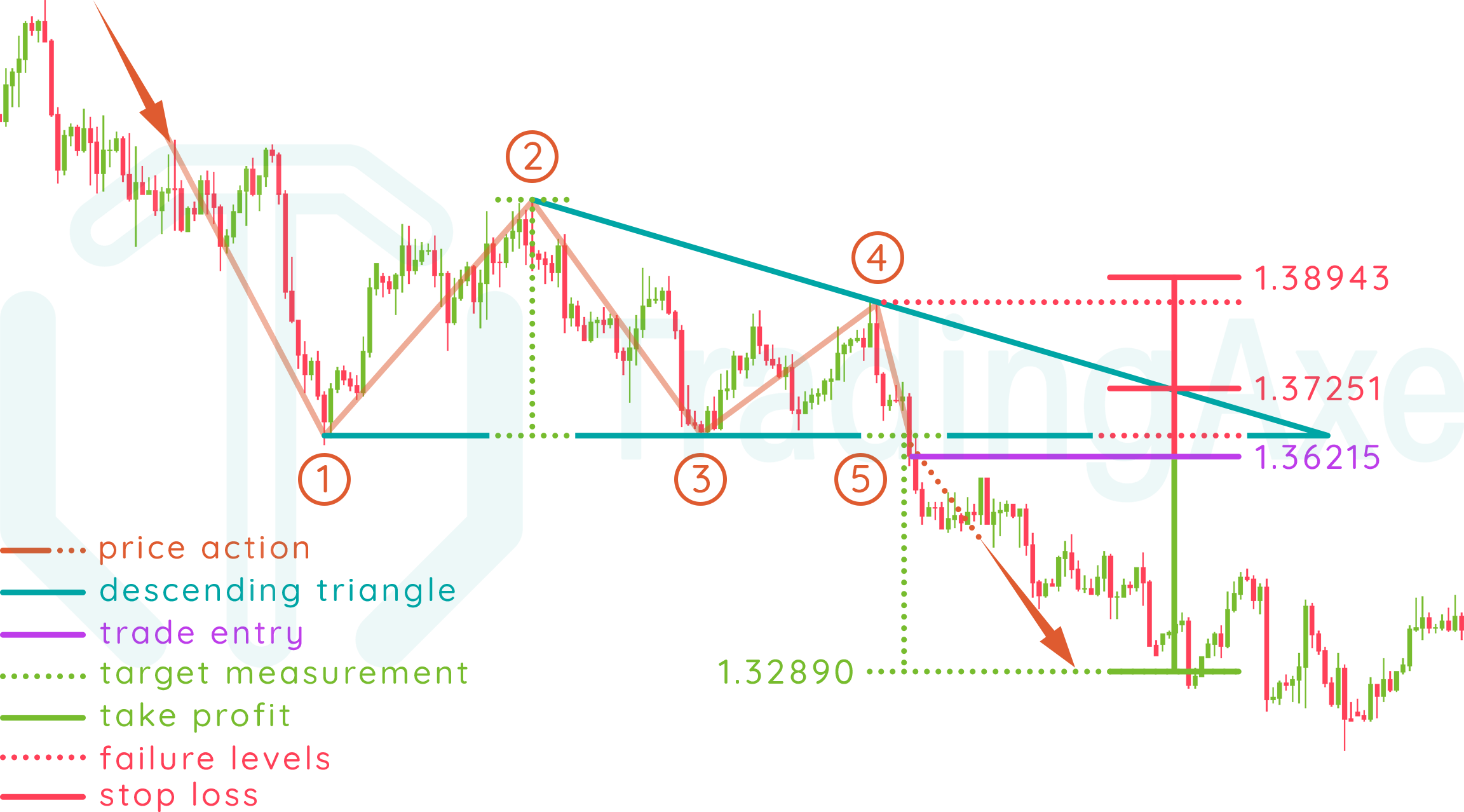### HOW TO TRADE DESCENDING TRIANGLE CHART PATTERN

Entry: after breaking the triangle's lower border at point (5), either with an entry after the breakout, or after a possible retest of the lower border.

Take profit: identified by measuring the vertical distance between the triangle's lower border and the first support (2), that measurement is then applied from the breakout rate (5)

Stop loss: can either be the triangle's lower border rate (5), or the last touch to the triangle's upper border before the breakout (4)

#### DESCENDING TRIANGLE PRICE ACTION

This chart pattern starts forming with bears already in control of the exchange rate's downtrend. Bulls make a stand at a certain rate and try holding it as much as they can, while bears keep squeezing on that rate till they finally break it and the exchange rate continues its downtrend.

Let's break down the pattern formation!

In a downtrend, price action finds the first resistance (1), which will be the horizontal resistance for the rest of the pattern formation.

Price action reverses direction from the first resistance (1) and goes upwards till it finds the first support (2), which will be the highest high in the pattern.

Price action reverses direction from the first support (2) and goes downwards, till it finds the second resistance (3), which will be around the rate of the first resistance (1)

Price action reverses direction from the second resistance (3) and goes upwards, till it finds the second support (4), which must be lower than the first support (2)

The pattern is completed when price action reverses direction from the second support (4) and goes downwards till it breaks the triangle's lower border at point (5)

#### NOTES ON DESCENDING TRIANGLE

Direction

Continuation

Type

Bearish

Occurrence

High

Common term

All

Before the breakout, 4 touches to the triangle's upper and lower borders are the minimum for a valid pattern, more touches are acceptable.

The breakout of the pattern is expected at around (half / two thirds) the triangle formation, measured from the first touch (1) to the intersection point of upper and lower borders.

The most common direction of the pattern is a continuation, but that doesn't rule out the existence of reversal descending triangles. The target measurement in that case will be applied from the upper border's breakout rate.

Volume usually decreases as the pattern is being formed, and increases when breaking or retesting the triangle's lower border (5)

This pattern is commonly found on all time frames.

#### DESCENDING TRIANGLE REWARD:RISK

When using the triangle's lower border rate as stop loss, R:R will depend on the (breakout rate-entry rate) distance, compared to the target measurement (1-2) distance.

When using the last touch before the breakout (4) as stop loss, the pattern's R:R improves when that touch is near the end of the triangle, as well as when the upper border's down-slope is sharper.

Always remember that both stop loss levels explained above are absolute, the actual stop loss rate for your trade setup should be a bit beyond those levels to give the trade setup some room to breathe, and of course, calculations for position size and R:R should be done with respect to those rates.

### DESCENDING TRIANGLE REAL TRADEForex chart - Descending triangle real trading example, as found on the H4 chart of USD/CAD using FXCM's MT4 platform

#### PRE-BREAKOUT CALCULATIONS

Since a descending triangle chart pattern can be spotted after the reversal from point (4), you can save yourself precious time by doing the following set of calculations before the breakout, since they don't rely on the trade's entry rate.

Note: identification of the lower border rate is subjective since the rates of points (1) and (3) won't be exactly the same. We prefer choosing the point with the higher rate to keep the pattern compact, which in this trade is point (3)

• Pattern length (point (2) rate - lower border rate)
• (1.40158 - 1.36524) * 10000 = 363.4 pips
• Take profit rate (lower border rate - pattern length)
• 1.36524 - (363.4 / 10000) = 1.32890
• Stop loss #1 rate (lower border rate + 20% of pattern length)
• 1.36524 + ((20 * 363.4 / 100) / 10000) = 1.37251
• Stop loss #2 rate (point 4 rate + 10% of pattern length)
• 1.38580 + ((10 * 363.4 / 100) / 10000) = 1.38943

Choosing when to enter the trade after the triangle's lower border breakout is always left to your best judgement. In this trade, we chose to enter the market at the closing rate of the candle that broke the triangle's lower border, which was a strong bearish candle that closed on a lower rate than that of the previous two resistances at points (1) and (3), suggesting a real breakout for the triangle's lower border.

• Trade entry rate
• At the closing rate of the candle that broke the triangle's lower border at point 5: 1.36215
• Take profit in pips (entry rate - take profit rate)
• (1.36215 - 1.32890) * 10000 = 332.5 pips
• Stop loss #1 in pips (stop loss #1 rate - entry rate)
• (1.37251 - 1.36215) * 10000 = 103.6 pips
• Stop loss #1 R:R (take profit in pips / stop loss #1 in pips)
• 332.5 / 103.6 = 3.209
• Stop loss #2 in pips (stop loss #2 rate - entry rate)
• (1.38943 - 1.36215) * 10000 = 272.8 pips
• Stop loss #2 R:R (take profit in pips / stop loss #2 in pips)
• 332.5 / 272.8 = 1.219

Currency pair

Timeframe

H4

Breakout

25-Feb-2016

Platform

MT4

Broker

FXCM